• 文章目录交叉熵目标函数更陡峭Xavier initialization He initialization He init 考虑ReLU函数He init 考虑Leaky ReLU函数结束语参考资料 交叉熵目标函数更陡峭 在论文中给了一个图示，一定程度上...
文章目录交叉熵目标函数更陡峭Xavier initialization He initialization He init 考虑ReLU函数He init 考虑Leaky ReLU函数结束语参考资料
交叉熵目标函数更陡峭
在论文中给了一个图示，一定程度上说明了为什么Cross Entropy用的很多，效果很好。图中上面的曲面表示的是交叉熵代价函数，下面的曲面表示的是二次代价函数，W1和W2分别表示层与层之间的连接权值。)

在1986年 Rumelhart 已经发现：logistic function 或者叫 conditional log-likelihood function: -log P（y|x) 的效果比 quadratic cost function（平方代价函数）的效果好很多的，原因在于 quadratic cost function（平方代价函数）在训练过程中会出现更多的 plateaus（平坦区域）。文章给出了一个两个参数下的图，图中采用的是具有单隐层的神经网络，激活函数使用的是 tanh 函数，对于输入信号进行随机初始化，可以看到二次代价函数具有更多的 plateaus （平坦区域）。

Xavier initialization 
早期的参数初始化方法普遍是将数据和参数初始化为标准高斯分布（均值0方差1），但随着神经网络深度的增加，这方法并不能解决梯度消失问题。那么我们应该怎么通过初始化来缓解这个问题呢？
论文中首先给出了一个启发式的方法，想法是初始化值和神经元的输入个数有关：

We initialized the biases to be 0 and the weights Wij at each layer  with the following commonly used heuristic, where U[−a, a] is the  uniform distribution in the interval (−a, a) and n is the size of the previous layer (the number of columns of W)

$W \sim U[-\frac{1}{\sqrt n},\frac{1}{\sqrt n}]\\ Var(W) = \frac{1}{3n}$
上面式子中，$W \sim$表示其中的每一个元素$W_{ij}$都是符合一个均匀分布的。其中n表示本层的输入size，也就是上一层的输出size。我们知道随机变量在[a,b] 间的均匀分布的方差为
$Var= \frac{(b-a)^2}{12}$
所以得到权重参数的方差是1/3n。先记一下，后面分析会讲到。
为了便于研究，作者假设使用线性激活函数$f(x)$，且在零点导数$f'(0)=1$。实际上，作者研究的是参数的线性区域，可以认为是对任务的一个简化。
对于一层网络：
$f(\textbf x) = \sum_i^n w_ix_i + b$
输出的方差：
$Var(f(\textbf x)) = \sum_i^n Var(w_i x_i)$
其中每一项：
$Var(w_i x_i) = E[w_i]^2Var(x_i) + E[x_i]^2Var(w_i)+Var(w_i)Var(x_i)$
当我们假设输入和权重都是0均值时（目前有了BN之后，每一层的输入也较容易满足），即$E[x_i] = E[w_i] = 0$，上式可以简化为：
$Var(w_i x_i) =Var(w_i)Var(x_i)$
由于w和x独立同分布，那么输出的方差就是
$Var(f(\textbf x)) = n Var(w_i) Var(x_i)$
我们发现，输出的方差是和输入的方差是一个线性倍数关系。假设$z^i$是第$i$层的输入向量，$s^i$是第$i$层激活函数的输入，$f$表示激活函数
$\textbf s^i = \textbf z^i W^i + \textbf b^i \\ \textbf z^{i+1} = f(\textbf s^i)\\ \textbf s^{i+1} = \textbf z^{i+1} W^{i+1} + \textbf b^{i+1}$
可以得到：
对于一个多层的网络，某一层的方差可以用累积的形式表达：
$Var[z^i] = Var[x] \prod_{i'=0}^{i-1}n_{i'} Var[W^{i'}]$
求反向我们可以得到（假设$f'(s_k^i) \approx 1$，其中Cost表示Loss）：
$\frac{\partial Cost}{\partial s_k^i} = f'(s_k^i) W_{k,\cdot}^{i+1} \frac{\partial Cost}{\partial s^{i+1}} \\ \frac{\partial Cost}{\partial w_{l,k}^i} = z_l^i \frac{\partial Cost}{\partial s_k^i}$
其中$Var[W^{i'}]$表示第$i'$层的共享权重的方差，对于$d$层的网络（大饼博士注：下面第一个式子的变量的layer号，符号上和上面求$\frac{\partial Cost}{\partial s_k^i}$的式子，有一点歧义难看懂。主要上面是正向去写，在同一个层里面$S^i$和$W^{i+1}$是一对。而下面第一个式子，理解成同一个层里面输入以及参数都是对应的$i'$，通过堆叠$d$层，得到最初的输入$s^i$的梯度的方差）
$Var[\frac{\partial Cost}{\partial s^i}]=Var[\frac{\partial Cost}{\partial s^d}] \prod_{i'=i}^{d}n_{i'+1} Var[W^{i'}] \\ Var[\frac{\partial Cost}{\partial w^i}]= \prod_{i'=0}^{i-1}n_{i'} Var[W^{i'}] \prod_{i'=i}^{d-1}n_{i'+1} Var[W^{i'}] \times Var[x] Var[\frac{\partial Cost}{\partial s^d}]$
如果我们希望，神经网络在前向计算的时候，输入输出的方差都是一致的，即$\forall (i,i'), Var[z^i] = Var[z^{i'}]$，我们需要满足：
$\forall i, \quad n_i Var[W^i] = 1$
类似的，如果我们希望反向计算的输入输出方差也是一致的，$\forall (i,i'), Var[\frac{\partial Cost}{\partial s^i}] = Var[\frac{\partial Cost}{\partial s^{i'}}]$，需要满足：
$\forall i, \quad n_{i+1} Var[W^i] = 1$
一个层的输入输出一般不相同，作为折中
$\forall i, \quad Var[W^i] = \frac{2}{n_i + n_{i+1}}$
这个就是Xavier初始化算法，认为神经网络每一层的参数的方差需要满足的方差（均值=0）。（注：这里并没有假设是符合什么分布的，只是要求方差是这样，所以理论上我们可以采用任意分布，只要方差等于上面的公式即可）。
常见的，我们可以用高斯分布，或者均匀分布来生成随机参数（对于Xavier初始化方式，例如pytorch提供了uniform和normal两种：）。

如果是采用均匀分布，根据前面讲过的，随机变量在[a,b] 间的均匀分布的方差为$Var= {(b-a)^2}/{12}$，那么我们只要用下面分布来采样，就可以得到上面的方差。这个公式也是Xavier论文中给出的建议。

$W \sim U[-\frac{\sqrt 6}{\sqrt {n_j + n_{j+1}}},\frac{\sqrt 6}{\sqrt {n_j + n_{j+1}}}]$

如果是采用高斯分布，那么直接就：

$W \sim N(0, { \frac{2}{n_i + n_{i+1}}})$
补充一句,caffe的Xavier实现有三种选择：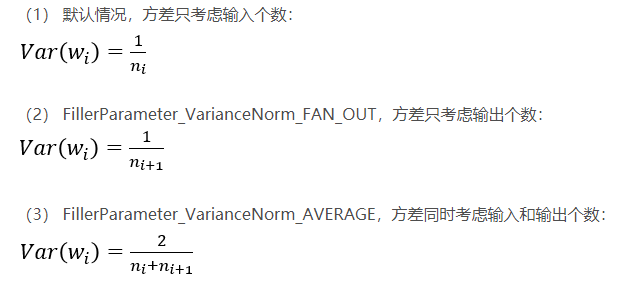He initialization 
前面Xavier方法只考虑了线性激活函数，而现在的神经网络（特别是CNN网络）采用的主要是ReLU和Leaky ReLU函数，He初始化方法就是来分析采用ReLU和Leaky ReLU激活函数下，如果我们希望每一层的输入输出方差不变，我们应该如何初始化权重参数，方法上和前一节基本一致。
对于一个卷积层或者全连接层，其表达式为
$y_l = W_l x_l + b_l.$
若$W_l$、$b_l$采样自0均值高斯分布，用$n_l$表示第$l$层$x_l$的维数，在卷积层，有$n_l=k^2c$，$k$为卷积核的边长，$c$为channel数，注意到$y_l$是$W_l$中元素与$x_l$中对应元素的乘积的和，则上式各变量的方差关系可以表示为：
$Var[y_l] = n_l Var[W_l x_l]=n_l Var[W_l]E[x_l^2].$
这里的$x_l$项前并不是其方差，而是$E[x^2_l]$，因为$x_l$通常并不具备0均值，例如ReLU激活函数得到的结果，$x_l$均为正值。注意到由于$W_l$是0均值的，所以无论$x_l$均值为多少，均有
$E[y_l]=E[W_l x_l]=0.$
He init 考虑ReLU函数
通过ReLU激活函数：$x_l=max(0,y_{l−1})$，仅正半轴有值，我们假设激活之前的值正负各一半，总体是对称的，可以得到：
$E[x_l^2] = \frac{1}{2} Var[y_{l-1}].$
因此（如果本文从上面看下来的话，看到这个公式就很熟悉了）：
$Var[y_l] = \frac{1}{2} n_l Var[W_l]Var[y_{l-1}].$
我们希望每一层的激活前值（卷积的结果，没有过激活函数）的方差一致，$Var[y_l]=Var[y_{l-1}]$，这个是和Xavier不一样的地方，这样就可以不考虑激活函数了。那么：
$\frac{1}{2} n_lVar[W_l] =1.$

如果是用高斯分布采样，$W \sim N(0,\frac{2}{n_l})$

如果是用均匀分布，那么：$W \sim U[-\sqrt{6/n_l},\sqrt{6/n_l}]$

He init 考虑Leaky ReLU函数
Leaky ReLU激活函数和导函数分别为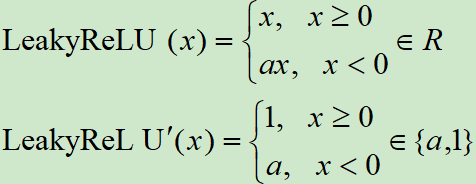所以：
$E[x_l^2] = \frac{1}{2}(1+\alpha^2) Var[y_l].$
类似上面的推导，可以得到，
$\frac{1}{2}(1+\alpha^2) n_lVar[W_l] =1.$

如果是用高斯分布采样，$W \sim N(0,\frac{2}{(1+\alpha^2) n_l})$

如果是用均匀分布，那么：$W \sim U[-\sqrt{6/(1+\alpha^2) n_l},\sqrt{6/(1+\alpha^2) n_l}]$

结束语
好了，到这里本篇就讲完了，介绍了Xavier与He intit的初始化方法，是非常常见的方法。后来还有很多工作来优化初始化，比如进一步考虑resnet网络结构时，有文章引入了Mean field，还有fixup initialization等方法。这些以后有机会再写了。最后说一句BN，因为可以强制要求每一层的数据符合0均值1方差，所以效果上和本文讨论的方法效果很类似。使得前向的数据会相对稳定。但是BN似乎并没有考虑反向梯度的稳定性，这一点目前更多是让resnet中的identity跳边来完成的，有这个identity跳边情况下，梯度至少在在跳边这一路上，可以identity透传，梯度就不容易消失。但是似乎梯度爆炸没有避免，什么情况下会爆炸呢？回看我们前面的分析，比如Xavier中，我们要求$\quad n_{i+1} Var[W^i] = 1$，反向就会稳定，如果>1，那么很显然，只要层数深，梯度就指数爆炸了，这样训练也会很难训。因此训练过程中的数据和梯度的稳定性是很重要的。
参考资料
 Understanding the difficulty of training deep feedforward neural networks
 Delving Deep into Rectifiers: Surpassing Human-Level Performance on ImageNet Classification
 https://blog.csdn.net/happynear/article/details/45440811
 https://blog.csdn.net/kangroger/article/details/61414426
 https://blog.csdn.net/qq_34784753/article/details/78668884
 https://blog.csdn.net/dss_dssssd/article/details/83959474
 https://blog.csdn.net/weixin_34221773/article/details/86085462
 https://www.cnblogs.com/itmorn/p/11132494.html#ct5


展开全文深度学习 神经网络
• 常用的初始化方法有四种，分别是高斯初始化、Xavier初始化和MSRA初始化。它们一般都把偏置初始化为0，但对权值进行随机初始化。其中，高斯初始化比较容易理解，就是根据某个高斯分布来初始化权值，但均值通常选0，...
在训练神经网络之前，必须对其全值和偏置进行初始化。常用的初始化方法有四种，分别是高斯初始化、Xavier初始化和MSRA初始化。它们一般都把偏置初始化为0，但对权值进行随机初始化。其中，高斯初始化比较容易理解，就是根据某个高斯分布来初始化权值，但均值通常选0，方差需要按经验人工选择。下面对Xavier和MSRA以及He做进一步的说明。

Xavier的基本思想是保持信息在神经网络中流动过程的方差不变。假设激活函数是关于0点对称的，且主要针对于全连接神经网络，适应于tanh和softsign。这种方法在实际应用时根据一个均匀分布来初始化权值。如果某个神经元 有  个输入  ,相应的连接权值为  ，则满足关系：

那么采用Xavier进行初始化的方法就是：

MSRA的基本思想与Xavier类似，但主要是针对ReLU和PReLU激活函数来设计的。这种方法在实际应用时根据一个方差为 的零均匀高斯分布来初始化权值，即

He的基本思想是正向传播时，状态值的方差保持不变；反向传播时，关于激活值的梯度的方差保持不变。

适用于ReLU的初始化方法：

适用于Leakly ReLU的初始化方法：

在darknet中CNN的权值初始化采用He的方法，具体实现见源码。后续我们会在【darknet源码解析】系列中详细讲解其实现。

完，

展开全文• 深度学习——Xavier初始化方法详细介绍，本文介绍一下深度学习参数初始化问题中耳熟能详的参数初始化方法——Xavier初始化。
个人微信公众号：AI研习图书馆，欢迎关注~
深度学习知识及资源分享，学习交流，共同进步~
1.引言
本文介绍一下深度学习参数初始化问题中耳熟能详的参数初始化方法——Xavier初始化。
文章：Understanding the difficulty of training deep feedforward neural networks
下载地址：https://machinelearning.wustl.edu/mlpapers/paper_files/AISTATS2010_GlorotB10.pdf
“Xavier”初始化方法是一种很有效的神经网络初始化方法，方法来源于2010年的一篇论文《Understanding the difficulty of training deep feedforward neural networks》。
通常，一般的深度学习文章上来就是实验，告诉读者这个实验结果好，然后由实验结果再反向给出一些无从验证的可能对可能不对的原因。而这篇文章虽然整体来看比较简单，但结构非常严谨：首先通过实验分析标准初始化方法的问题；然后根据两个目标——状态方差和梯度方差保持不变推导出参数的特点，给出Xavier初始化方法的具体形式；最后通过实验验证Xavier初始化的效果确实不错。
在开始阅读下面的内容之前，我们需要牢记参数初始化的目的是为了让神经网络在训练过程中学习到有用的信息，这意味着参数梯度不应该为0。而我们知道在全连接的神经网络中，参数梯度和反向传播得到的状态梯度以及入激活值有关——激活值饱和会导致该层状态梯度信息为0，然后导致下面所有层的参数梯度为0；入激活值为0会导致对应参数梯度为0。所以如果要保证参数梯度不等于0，那么参数初始化应该使得各层激活值不会出现饱和现象且激活值不为0。我们把这两个条件总结为参数初始化条件：

初始化必要条件一：各层激活值不会出现饱和现象。
初始化必要条件二：各层激活值不为0。

需要说明的是这篇论文只研究了解决分类问题的5层的全连接神经网络，每个隐层的神经元个数为1000，并且仅探讨了三种激活函数：sigmoid、tanh、softsign。
2.标准初始化方法
形式
把权重矩阵初始化为如下形式：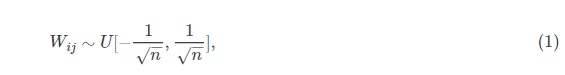其中U[−a,a]是区间(−a,a)上的均匀分布，n是入神经网络的大小。易知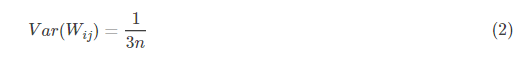在深入介绍标准初始化之前，首先给出随机变量方差的两个性质：

假设随机变量X和随机变量Y相互独立，则有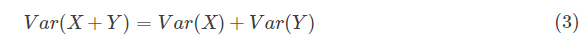假设随机变量X和随机变量Y相互独立，且E(X)=E(Y)=0，则有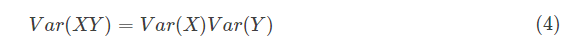神经网络的隐层状态计算表达式：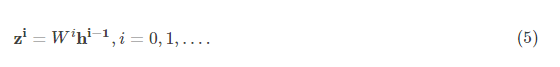其中激活值计算表达式：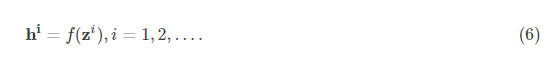论文中的符号和我的有些区别：论文中用zz表示激活值，ss表示状态值。我这里按自己的习惯来写。
现在把输入xx的每一维度xx看做一个随机变量，并且假设E(x)=0E(x)=0，Var(x)=1Var(x)=1。假设WW和xx相互独立，则隐层状态的方差为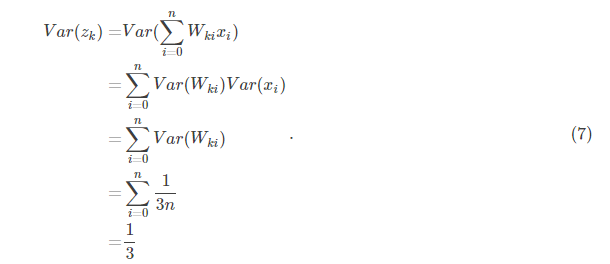可以看出标准初始化方法得到一个非常好的特性：隐层的状态的均值为0，方差为常量1313，和网络的层数无关，这意味着对于sigmoid函数来说，自变量落在有梯度的范围内。
但是因为sigmoid激活值都是大于0的，会导致下一层的输入不满足E(⋅)=0E(⋅)=0。其实标准初始化也只适用于满足下面将要提到的Glorot假设的激活函数，比如tanh。
训练过程中的激活值特性
作者探究了三种激活函数对应的神经网络在训练过程中各层激活值的分布情况，得到下面的结果图。

sigmoid神经网络在每层的激活值均值和标准偏差在训练过程中的演变情况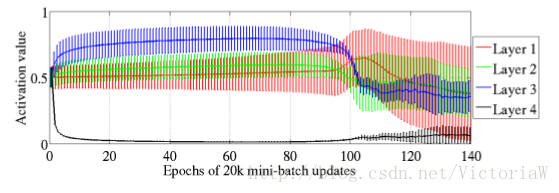这幅图比较有意思的是Layer 4的激活值在训练开始没多久就变成0（均值为0并且方差为0说明大多数激活值都为0），即进入饱和状态；而在最后又慢慢跳出饱和状态。
为什么会出现这种现象呢？读者可以自己想一想。在原论文中有作者给的解释。
tanh神经网络和sofsign神经网络在训练过程中每层激活值的分布情况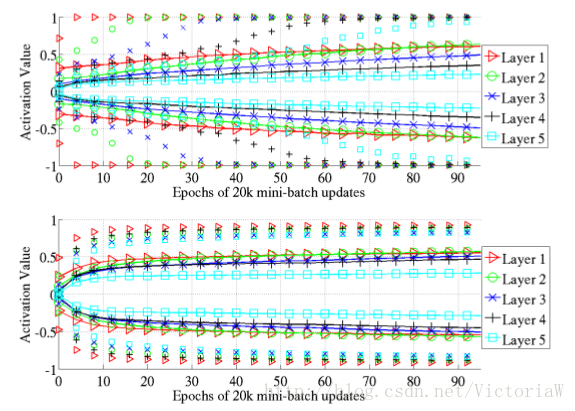可以看到在tanh神经网络中第一层最先饱和，然后是后面各层按顺序出现饱和。softsign神经网络中饱和现象并不严重，而且各层差不多同时出现饱和情况。
作者提到出现这种现象的原因有待于探究。
训练完成后的激活值特性
训练完成后tanh神经网络和softsign神经网络各层的激活值的直方图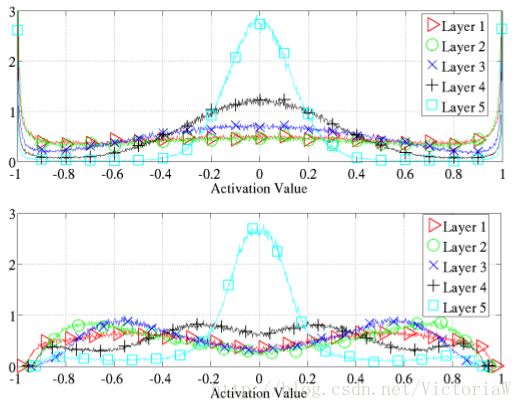tanh神经网络的低层出现了饱和现象：-1和1对应的神经元非常多。
3. Xavier初始化
在文章开始部分我们给出了参数初始化的必要条件。但是这两个条件只保证了训练过程中可以学到有用的信息——参数梯度不为0。而Glorot认为：优秀的初始化应该使得各层的激活值和状态梯度的方差在传播过程中的方差保持一致：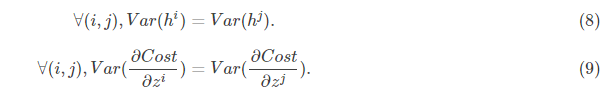我们把这两个条件称为Glorot条件。
形式
在某些假设下反向传播梯度和参数梯度表达式
首先给出关于状态的梯度和关于参数的梯度的表达式：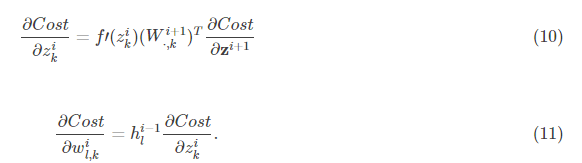为了使得网络中信息更好的流动，每一层输出的方差应该尽量相等。
基于这个目标，现在我们就去推导一下：每一层的权重应该满足哪种条件。
文章先假设的是线性激活函数，而且满足0点处导数为1，即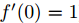现在我们先来分析一层卷积：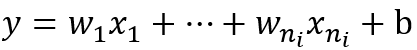其中ni表示输入个数。
根据概率统计知识我们有下面的方差公式：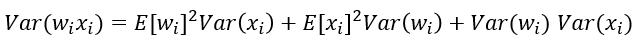特别的，当我们假设输入和权重都是0均值时（目前有了BN之后，这一点也较容易满足），上式可以简化为：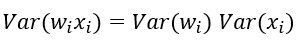进一步假设输入x和权重w独立同分布，则有：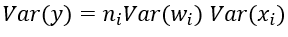于是，为了保证输入与输出方差一致，则应该有：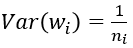对于一个多层的网络，某一层的方差可以用累积的形式表达：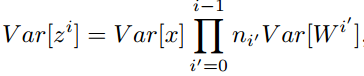特别的，反向传播计算梯度时同样具有类似的形式：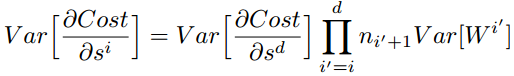综上，为了保证前向传播和反向传播时每一层的方差一致，应满足：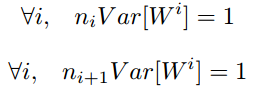但是，实际当中输入与输出的个数往往不相等，于是为了均衡考量，最终我们的权重方差应满足：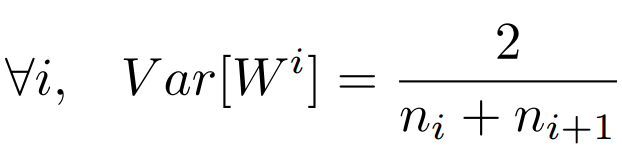学过概率统计的都知道 [a,b] 间的均匀分布的方差为：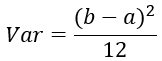因此，Xavier初始化的实现就是下面的均匀分布：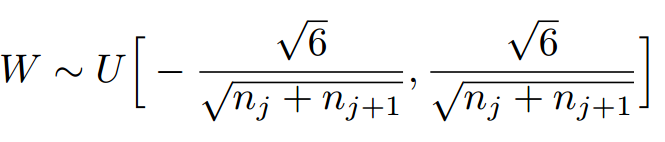4. caffe中的具体实现
下面，我们来看一下caffe中具体是怎样实现的，代码位于include/caffe/filler.hpp文件中。
template <typename Dtype>
class XavierFiller : public Filler<Dtype> {
public:
explicit XavierFiller(const FillerParameter& param)
: Filler<Dtype>(param) {}
virtual void Fill(Blob<Dtype>* blob) {
CHECK(blob->count());
int fan_in = blob->count() / blob->num();
int fan_out = blob->count() / blob->channels();
Dtype n = fan_in;  // default to fan_in
if (this->filler_param_.variance_norm() ==
FillerParameter_VarianceNorm_AVERAGE) {
n = (fan_in + fan_out) / Dtype(2);
} else if (this->filler_param_.variance_norm() ==
FillerParameter_VarianceNorm_FAN_OUT) {
n = fan_out;
}
Dtype scale = sqrt(Dtype(3) / n);
caffe_rng_uniform<Dtype>(blob->count(), -scale, scale,
blob->mutable_cpu_data());
CHECK_EQ(this->filler_param_.sparse(), -1)
<< "Sparsity not supported by this Filler.";
}
};

由上面可以看出，caffe的Xavier实现有三种选择
（1） 默认情况，方差只考虑输入个数：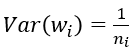（2） FillerParameter_VarianceNorm_FAN_OUT，方差只考虑输出个数：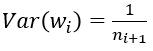（3） FillerParameter_VarianceNorm_AVERAGE，方差同时考虑输入和输出个数：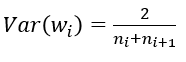之所以默认只考虑输入，个人觉得是因为前向信息的传播更重要一些。
5.    Xavier初始化的缺点
因为Xavier的推导过程是基于几个假设的，其中一个是激活函数是线性的。这并不适用于ReLU激活函数。另一个是激活值关于0对称，这个不适用于sigmoid函数和ReLU函数。所以可以看到图11中并没有对sogmoid网络应用Xavier初始化。
可以实验验证sigmoid激活函数用Xavier初始化后的初始化激活值、反向梯度、参数梯度特性：以MNIST做训练数据，发现标准初始化和Xavier初始化得到的初始激活、参数梯度特性是一样的。激活值的方差逐层递减，参数梯度的方差也逐层递减。


展开全文参数初始化
• 传统的初始化的方法是使用固定标准差的高斯分布，在caffe中使用的是经典的Xavier初始化方法，当然直接进行预训练也是初始化的一种好的方法，一个好的初始化可以有助于收敛到一个优秀的局部最优解，且收敛速度快。...
神经网络权值初始化方法

引言
神经网络权值的初始化对于网络训练速度以及收敛性有很大影响，因此我们需要合适的方法。传统的初始化的方法是使用固定标准差的高斯分布，在caffe中使用的是经典的Xavier初始化方法，当然直接进行预训练也是初始化的一种好的方法，一个好的初始化可以有助于收敛到一个优秀的局部最优解，且收敛速度快。但这些方法在ReLU系列以及网络层数较深时，这样的初始化无法得到很好的结果。何凯明大佬提出了MSRA初始化。MSRA初始化可以说是专门为ReLU系列激活函数特意设计的。具体可参考文章《Delving Deep into Rectifiers:Surpassing Human-Level Performance on ImageNet Classification》

Xavier初始化
可以参考这两篇文章，这两位讲的很详细
(1).https://blog.csdn.net/weixin_35479108/article/details/90694800
(2).深度前馈网络与Xavier初始化原理 - 夕小瑶的文章 - 知乎
https://zhuanlan.zhihu.com/p/27919794

MSRA初始化
可以参考这两篇文章，这两位讲的很详细
(1).Delving Deep into Rectifiers - fountain-k的文章 - 知乎
https://zhuanlan.zhihu.com/p/103825243
(2).https://blog.csdn.net/happynear/article/details/45440811
同时这种初始化方法通过前向传递初始化或者反向传递初始化都是可以的


展开全文• 装载自：https://blog.csdn.net/shuzfan/article/details/51338178 “Xavier初始化方法是一种很有效的神经网络初始化方法，方法来源于2010年的一篇论文《Understanding the difficulty...
• 文章目录标准初始化方法形式激活值和梯度特性实验Xavier初始化形式满足Glorot条件的初始化激活值和梯度特性实验Xavier初始化的缺点 Understanding the difficulty of training deep feedforward neural networks by ...深度学习
• 初始化方法： 假设激活函数关于0对称，且主要针对于全连接神经网络。适用于tanh和softsign 论文地址：Understanding the difficulty of training deep feedforward neural networks 参考资料：深度学习之参数...
• 初始化权值参数 选择一个合适的梯度下降算法（例如：Adam，RMSprop等） 重复下面的迭代过程： 输入的正向传播 计算loss function 的值 反向传播，计算loss function 相对于权值参数的梯度值 根据选择的梯度...
• DL之DNN优化技术：DNN中权重初始值优化【Xavier初始值】的简介、使用方法详细攻略 目录 【Xavier初始值】的简介 【Xavier初始值】的使用方法Xavier初始值】的简介 Xavier Glorot等人的论文中推荐...
• Xavier初始化方法是一种很有效的神经网络初始化方法，方法来源于2010年的一篇论文《Understanding the difficulty of training deep feedforward neural networks》，可惜直到近两年，这个方法才逐渐得到更多人...深度学习
• 首先介绍一下Xavier初始化方法比直接用高斯分布进行初始化W的优势所在：  一般的神经网络在前向传播时神经元输出值的方差会不断增大,而使用Xavier等方法理论上可以保证每层神经元输入输出方差一致。  这里先介绍...
• 本文主要详解两种神经网络初始化方法及如何使用Pytorch实现网络初始化 Xavier 初始化 对于神经网络中的layer ilayer \ ilayer i，假设它的输入是ziz^izi，si=ziWi+bis^i=z^iW^i+b^isi=ziWi+bi，激活...神经网络 深度学习
• ## 深度学习——Xavier初始化方法

万次阅读 多人点赞 2016-05-07 18:39:44
Xavier初始化方法是一种很有效的神经网络初始化方法，方法来源于2010年的一篇论文《Understanding the difficulty of training deep feedforward neural networks》，可惜直到近两年，这个方法才逐渐得到更多人...
• Xavier初始化方法 源自论文：Understanding the difficulty of training deep feedforward neural networks Xavier;Bengio 思想：正向传播时，每层激活值的均值为0，方差相同，反向传播时，每层状态值的梯度的...
• Pytorch权重初始化方法——Kaiming、Xavier 结论 结论写在前。Pytorch线性层采取的默认初始化方式是Kaiming初始化，这是由我国计算机视觉领域专家何恺明提出的。我的探究主要包括： 为什么采取Kaiming初始化？ 考察...
• 早期的参数初始化方法普遍是将数据和参数normalize为高斯分布（均值0方差1），但随着神经网络深度的增加，这方法并不能解决梯度消失问题。 Xavier初始化的作者，Xavier Glorot，在Understanding the difficulty ...
• Xavier[发音 ‘zeɪvɪr]初始化方法是深度学习中比较常用和有效的一种权重初始化方法，其名字是以其发明者命名的。 它的基本设计原则是为了使网络中的信息更好地流动，网络各层激活值及状态梯度的方差应尽量保持...
• 基础知识 首先介绍一下Xavier初始化方法比直接用高斯分布进行初始化W的优势所在： 一般的神经网络在前向传播时神经元输出值的方差...
• 神经网络中权值初始化方法 《Understanding the difficulty of training deep feedforward neural networks》 可惜直到近两年，这个方法才逐渐得到更多人的应用和认可。 为了使得网络中信息更好的流动，每一...
• 初始化方法由Bengio等人在2010年的论文《Understanding the difficulty of training deep feedforward neural networks》中提出。 它为了保证前向传播和反向传播时每一层的方差一致，根据每层的输入个数和输出个数...
• 参数初始化就是这么一个容易被忽视的重要因素，因为不仅使用者对其重要性缺乏概念，而且这些操作都被TF、pytorch这些框架封装了，你可能不知道的是，糟糕的参数初始化是会阻碍复杂... 早期的参数初始化方法普遍是将......